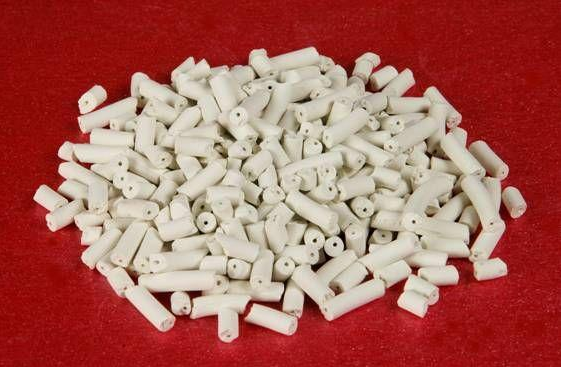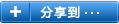# 新疆脱硫剂的选择

### 发布日期：2015-10-15 00:00 来源：http://www.xjjyhy.com 点击：

1、钙法脱硫

CaO+H2O=Ca(OH)2，Ca(OH)2=Ca2++2OH-，使两种溶液进行化学反应吸收溶解的SO2：

Ca2++SO32-=CaSO3，CaSO3+(1/2)H2O=CaSO3·(1/2)H2O。

HSO3-+(1/2)O2=H++SO42-，Ca2++SO42-=CaSO4↓，CaSO4+2H2O=CaSO4·2H2O↓

Z后的产物是CaSO4·2H2O↓（就是石膏），是一种不溶于水的稳定物质，可用于建筑材料和水泥制作的原料。

2、镁法脱硫

Mg(OH)2+SO2=MgSO3+H2O，

Mg(HCO3)2+SO2=MgSO3+H2O+2CO2，

MgSO3+H2O+SO2=Mg(HSO3)2，

MgO+Mg(HSO3)2=2MgSO3+H2O 要完成此过程MgO要有5%的过量。

MgSO3+1/2O2=MgSO4，

MgO+SO2+1/2O2=MgSO4在经过结晶浓缩可生产肥料级的MgSO4·1H2O，也可生产工业级MgSO4·7HO，如果不进行或抑制MgSO3氧化，可以再生新疆脱硫剂并生产硫酸。

3、氨法脱硫

H2SO4+2NH3=(NH4)2SO4，HNO3+NH3=NH4NO3，

SO2+H2O+1/2O2=H2SO4，H2SO3+2NH3=(NH4)2SO3，

(NH4)2SO3+H2O+SO2=2NH4HSO3，NH4HSO3+NH3=(NH4)2SO3。

(NH4)2SO3在数小时内可以被大气氧化：(NH4)2SO3+1/2O2=(NH4)2SO4氨法的优势是用量少、二次污染少、副产品用途广泛（可以作为氨肥）、也可以实现循环再生使用、脱硫、脱硝一次完成，但是氨水的价格高1200—1500元/吨，配套设备要求高，一次性投资大。

4、钠法脱硫

2NaOH+SO2=Na2SO3+H2O，

Na2CO3+SO2=Na2SO3+CO2↑，

Na2SO3+SO2+H2O=2NaHSO3，

NaHSO3+NaOH=Na2SO3+H2O，

2NaHSO3+Na2CO3=2Na2SO3+H2O+CO2↑

5、炭法脱硫### 相关标签：新疆脱硫剂

#### Z近浏览：### 扫描二维码